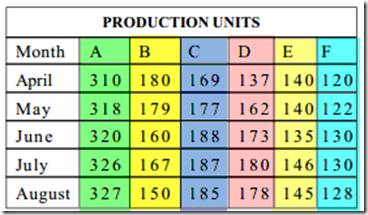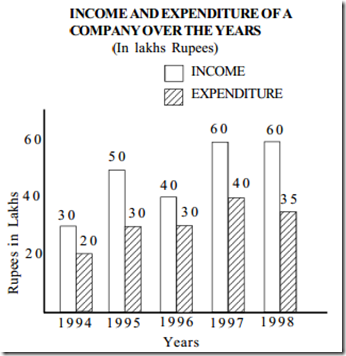## Wednesday, 14 October 2015

Dear Reader .. We are Providing Questions and Answers for PO Practice Purpose. check below Data Interpretation Section .
I. Directions (Qs. 1-5) study the following table and answer the questions given
below it. Production of sugar by six major production units of India in Million Tonnes1. in Which month the Unit B has a Contribution of Approximately 15 % in the Total Sugar production ?
A. August
B. June
C. July
D. April
2. Which of the following Units  Shows Continues Increase in Production of Sugar over months
A.   A
B.   B
C.   C
D.   D
3. In the case of Unit E, in which of the Following pairs for months the production of Sugar was equal ?
A. April & June
B. June & July
C. July & August
D. April & may
4. In the month of June ,How many Units have a share of More than 25% of the total Production of Sugar ?
A. One
B. Three
C. Two
D. Four
5. what was Approximate percentage decrease in sugar Production of Unit B in June as Compared to April ?
A. 8%
B. 10%
C. 15%
D. 18%
1. C   2. A  3. D  4. A 5. B
Directions (6-10 ) Study the following graph and Answer the Questions Given Below6. what was the difference in profit between 1995 and 1996 ?
A. Rs. 10 lakhs
B. Rs. 5 lakhs
C. Rs. 15 lakhs
D. No profit
7. in the case of how many years was the income more than the average income of given years ?
A. one
B. two
C. three
D. four
8. what was the percentage increase in Expenditure from 1996 to 1997 ?
A. 10
B. (33)1/3
C. (66)2/3
D. 20
9. The income in 1996 was equal to the Expenditure of which of the following Year ?
A. 1994
B. 1995
C. 1997
D. 1998
10. in which of the following year was the profit the maximum ?
A. 1994
B. 1995
C. 1996
D. 1998